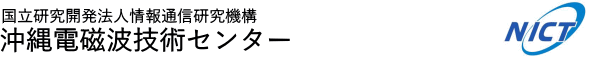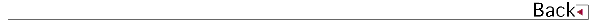# 2007\$BG/EY(B (2007.04\$B!A(B2008.03)

## \$B8&5fO@J8(B

• \$B;T@n!!9a(B, \$BEO7D>2,(B \$B7z;V(B, \$B;yEg(B \$B@50lO:(B, \$BF#0f(B \$BCR;K(B, Kuroshio variations in the upstream region as seen by HF radar and satellite altimetry data, International Journal of Remote Sensing, 2007.
• D. Pancheva, P. Mukhtarov, N. J. Mitchell, B. Andonov, E. Merzlyakov, W. Singer, \$BB<;3(B \$BBY7<(B, \$B@nB<(B \$B@?<#(B, J. Xiong, W. Wan, W. Hocking, D. Fritts, D. Riggin, C. Meek, A. Manson, Latitudinal wave coupling of the stratosphere and mesosphere during the major stratospheric warming in 2003/2004, ANNALES GEOPHYSICAE, Vol.26, P467-483, 2008.

## \$B3X=Q2r@b(B

• \$B:4F#(B \$B?82p(B, \$BF|K\\$K\$*\$1\$k5\$>]%l!<%@!<\$NH/E8(B, Development of weather radar in Japan, \$BF|K\5\$>]3X2q(B \$B3X2q;o!VE75\$!W(B, 2007,Vol.54, No.9, P17-20.

## \$B<}O?O@J8(B

• \$B:4C](B \$B@?(B, \$B>>2,(B \$B7z;V(B, \$BG_86(B \$B=SI'(B, \$BFg0f(B \$B>O;L(B, \$B1:DM(B \$B@6Jv(B, \$BJ!CO(B \$B0l(B, Polarimetric calibration experiment of ALOS PALSAR with polarization-selective dihedrals, 2007 IEEE International Geoscience and Remote Sensing Symposium (IGARSS 2007), 2007, P1596-1598.
• \$BCf@n(B \$B>!9-(B, \$B=P@\$(B \$B\$f\$+\$j(B, \$BKLB<(B \$B9/;J(B, Robert MENEGHINI, "Characteristics of vertical profile of rainfall parameters in Baiu frontal rainfall using 2D-Video distrometer, COBRA, and 400MHz Wind Profiler", AMS 33rd Radar Conference, 2007, P10.6, 1-4.
• \$B;yEg(B \$B@50lO:(B, \$B
• \$BJ!CO!!0l(B, \$B8EC+!!M'Gn(B, \$BLnED!!1Q0l(B, \$B:4C](B \$B@?(B, New Polarimetric Calibration Proposal and Its Evaluation Using ALOS PALSAR Calibration Campaign Measurements, 2007 IEEE International Geoscience and Remote Sensing Symposium (IGARSS 1007), P1610, 1-3, 2007.
• \$BFg0f(B \$B>O;L(B, \$BG_86(B \$B=SI'(B, \$B>>2,(B \$B7z;V(B, \$B:4C](B \$B@?(B, \$B1:DM(B \$B@6Jv(B, Dependency analysis of normalized radar cross section of ocean surface on ocean winds using an airborne dual-frequency polarimetric SAR, IEEE International Geoscience and Remote Sensing Symposium 2007, We02AF, P1-4, 2007.
• \$B:4C](B \$B@?(B, \$B>>2,(B \$B7z;V(B, \$BG_86(B \$B=SI'(B, \$BFg0f(B \$B>O;L(B, \$B1:DM(B \$B@6Jv(B, Polarization-Selective Dihedrals and Its Application to Polarimetric Calibration Experiment of ALOS PALSAR, 2007 International Symposium on Antennas and Propagation, ISAP2007, No.3D1-4, P780-783, 2007.

## \$B0lHL8}F,H/I=(B

• \$B:4C](B \$B@?(B, \$B=P@\$(B \$B\$f\$+\$j(B, \$BCf@n(B \$B>!9-(B, \$B;yEg(B \$B@50lO:(B, \$B:4F#(B \$B?82p(B, C-band\$B2-FlJPGH9_1+%l!<%@\$K\$h\$k3\$LL4QB,(B, Ocean surface observation by C-band polarimetric weather radar in Okinawa, \$BF|K\%j%b!<%H%;%s%7%s%03X2q(B \$BBh(B42\$B2s3X=Q9V1i2q(B, 2007, B10, P89-90.
• \$B:4F#(B \$B?82p(B, \$B1R@1?dDj9_1+NL\$HCO>e1+NL7W%G!<%?\$H\$NHf3S8!>Z(B, JEPP\$B>>K\J?@.(B19\$BG/EYBh(B1\$B2s8&5f0Q0w2q(B, 2007.
• \$BHx>e(B \$BK|N\$;R!\$>eED(B \$BGn!\$=P@\$(B \$B\$f\$+\$j!\$G_1+A0@~BS\$K\$*\$1\$kBPN.@-9_?e\$NCO>e1+E)N37BJ,I[\$NFCD'!\$(B2007, \$BF|K\5\$>]3X2q(B \$B=U5(Bg2q!\$(BP367.
• \$B:4F#(B \$B?82p(B, \$B=P@\$(B \$B\$f\$+\$j(B, \$BCf@n(B \$B>!9-(B, \$B:4C](B \$B@?(B, \$B%I%C%W%i!<5\$>]%l!<%@!<\$K\$h\$kFMIw8!=P\$K4X\$9\$k=tLdBj(B, \$BF|K\5\$>]3X2q(B2007\$BG/EY=U4|Bg2q(B, 2007, B207.
• \$B=P@\$(B \$B\$f\$+\$j(B, \$B9b66(B \$BD*9((B, \$BCf@n(B \$B>!9-(B, \$B:4F#(B \$B?82p(B, \$B0f8}(B \$B=SIW(B, COBRA\$B4QB,\$K\$h\$kBPN.@-9_?e0h\$NM;2rAX9bEY(B -\$B2-FlCOJ}\$NG_1+A0@~;vNc\$K\$D\$\$\$F(B-, \$BF|K\5\$>]3X2q(B \$B=U5(Bg2q(B, 2007, P100.
• \$B;yEg(B \$B@50lO:(B, \$B:4F#(B \$B7r<#(B, \$B
• \$B
• \$B
• \$B:4F#(B \$BM[2p(B, \$BCfB<(B \$BBn;J(B, \$BDEED(B \$BIRN4(B, \$B:4F#(B \$B?82p(B, \$BB<;3(B \$BBY7<(B, \$B:4C](B \$B@?(B, \$B>.7?%i%^%s%i%\$%@!<\$K\$h\$k2-Fl0!G.BS0h2x5\$J,I[\$N4QB,(B, Observation of water vapor distribution in the lower troposphere in the sub-tropical region of Okinawa by portable Raman lidars, \$BCO5eEE<'5\$!&CO5eOG@17w3X2q(B \$BBh(B122\$B2s9V1i2q(B, 2007, B005-04.
• \$B:4F#(B \$BM[2p(B, \$BCfB<(B \$BBn;J(B, \$BDEED(B \$BIRN4(B, \$B:4F#(B \$B?82p(B, \$BB<;3(B \$BBY7<(B, \$B:4C](B \$B@?(B, \$B>.7?%i%^%s%i%\$%@!<\$K\$h\$k2-Fl0!G.BS0h2x5\$J,I[\$N4QB,(B, \$BF|K\5\$>]3X2q(B \$B=)5(Bg2q(B, 2007, P110, 362.
• \$B=P@\$(B \$B\$f\$+\$j(B, \$B:4C](B \$B@?(B, \$B:4F#(B \$B?82p(B, \$B9b66(B \$BD*9((B, \$B2VEZ(B \$B90(B, \$B0f8}(B \$B=SIW(B, COBRA\$B4QB,\$K\$h\$kBfIw(B0704\$B9f\$N4c\$NJI1@\$N9=B\$(B, \$BF|K\5\$>]3X2q(B2007\$BG/EY=)4|Bg2q(B, 2007, C261, 232.
• \$B:4F#(B \$B?82p(B, \$B=P@\$(B \$B\$f\$+\$j(B, \$BCf@n(B \$B>!9-(B, \$B:4C](B \$B@?(B, \$BJPGH%I%C%W%i!<%l!<%@!<\$G4QB,\$5\$l\$?J#?t\$N%a%=%5%\$%/%m%s(B, \$BF|K\5\$>]3X2q(B \$B=)5(Bg2q(B, 2007, C255.
• \$B:4C](B \$B@?(B, \$BEEGH\$K\$D\$\$\$F(B, \$BLnDl>.3X9;%5%\$%(%s%965<
• \$B;T@n(B \$B9a(B, \$BEO7D
• \$B:4F#(B \$B?82p(B, \$B1R@1?dDj9_1+NL\$HCO>e1+NL7W%G!<%?\$H\$NHf3S8!>Z(B, JEPP\$B>>K\J?@.(B19\$BG/EYBh(B2\$B2s8&5f0Q0w2q(B, 2007.
• \$B:4F#(B \$B?82p(B, East Asian Monsoon Study using Remote-sensing Observation from Okinawa and Satellite, Japan-Taiwan Joint Workshop for the EAMEX and MAHASRI, 2007.
• \$B
• \$B:4C](B \$B@?(B, \$B2-Fl\$NIw!&1+!&3\$\$r8+pJsDL?.8&5f5!9=Bg59L#Bg5\$4QB,;\@_\$N8+3X2q\$*\$h\$S2;GH\$rMQ\$\$\$?
• \$B:4F#(B \$B?82p(B, \$B1R@1?dDj9_1+NL\$HCO>e1+NL7W%G!<%?\$H\$NHf3S8!>Z(B, JEPP\$B>>K\!V4QB,%W%i%s!W@.2LJs9p2q(B, 2008.
• \$B:4F#(B \$B?82p(B, \$BN&>e9_?e?dDj@:EY\$N8=>u\$HLdBjE@(B, CREST-GSMaP\$B8x3+@.2LJs9p2q(B, 2008.
• \$BFg0f(B \$B>O;L(B, THE INFLUENCE OF SPATIAL CHANGE OF CURRENT FIELD ON CURRENT MEASUREMENT BY HF OCEAN SURFACE RADAR, Ocean Science Meeting 2008, 2008.
• \$B:4F#(B \$B?82p(B, \$B=P@\$(B \$B\$f\$+\$j(B, \$B:4C](B \$B@?(B, \$B5vED(B \$B@9Li(B, \$BNS(B \$B=S9((B, \$B:O5H(B \$B?.(B, COBRA\$B\$G4QB,\$5\$l\$?J#?t\$N%a%=%5%\$%/%m%s(B, \$BL>8E20Bg3X(BHyARC-NICT\$B2-Fl%;%s%?!<(B \$BJ?@.(B19\$BG/EY6&F18&5f=82q(B, 2008.
• \$B8EK\(B \$B=_0l(B, \$Bo4QB,(B, \$BL>8E20Bg3X(BHyARC-NICT\$B2-Fl%;%s%?!<(B \$BJ?@.(B19\$BG/EY6&F18&5f=82q(B, 2008.
• Nga, Pham Thi Thanh, \$BCfB<(B \$B7r<#(B, \$B8E_7(B \$BJ89>(B, \$B:4F#(B \$B?82p(B, \$BG_1+5(\$N2-Fl\$G\$N28E20Bg3X(BHyARC-NICT\$B2-Fl%;%s%?!<(B \$BJ?@.(B19\$BG/EY6&F18&5f=82q(B, 2008.
• \$B:4C](B \$B@?(B, \$B=P@\$\$f\$+\$j(B, \$BCf@n>!9-(B, \$B;yEg@50lO:(B, \$B2-FlJPGH9_1+%l!<%@(B(COBRA)\$B\$K\$h\$k3\$LL\$N;6MpFC@-7WB,(B, \$B2-Fl0!G.BS0h\$K\$*\$1\$k1@!&9_?e%7%9%F%`\$HBg5\$6-3&AX!"3\$MNI=AX\$N4QB,E*8&5f\$K4X\$9\$k6&F18&5f=82q(B, 2008, No.1115, P18-20.
• \$BCfB<(B \$B7r<#(B, "Nga, Pham Thi Thanh", \$B8E_7(B \$BJ89>(B, \$B:4F#(B \$B?82p(B, \$B2-Fl\$K\$*\$1\$kG_1+5(\$N2
• \$B8EK\(B \$B=_0l(B, \$BBg@>(B \$B@5E5(B, \$BDEED(B \$BIRN4(B, \$B:4F#(B \$B?82p(B, \$BB<;3(B \$BBY7<(B, \$B0f8}(B \$B=SIW(B, 400MHz\$BBS%&%#%s%I%W%m%U%!%\$%i!&(BRASS\$B4QB,\$K\$h\$k0!G.BS0h29EYHy:Y9=B\$\$N4QB,(B, \$BF|K\5\$>]3X2q(B2007\$BG/EY=U4|Bg2q(B, No.C460, P177, 2007.
• \$B1:DM(B \$B@6Jv(B, \$BG_86(B \$B=SI'(B, \$BFg0f(B \$B>O;L(B, \$B>>2,(B \$B7z;V(B, \$B:4C](B \$B@?(B, \$B>.NS(B \$BC#<#(B, \$B9R6u5!#S#A#R%7%9%F%`\$H\$=\$N1~MQ(B, \$BF|K\CO5eOG@12J3XO"9g(B2007\$BG/Bg2q(B, 2007.
• \$BFg0f(B \$B>O;L(B, \$BG_86(B \$B=SI'(B, \$B>>2,(B \$B7z;V(B, \$B:4C](B \$B@?(B, \$B1:DM(B \$B@6Jv(B, \$B9R6u5!Ek:\(B2\$B<~GH%]%i%j%a%H%j%C%/9g@.3+8}%l!<%@\$K\$h\$k3\$>eIw7WB,(B(Measurement of ocean surface wind by using airborne dual-frequency polarimetric synthetic aperture radar), \$BF|K\CO5eOG@12J3XO"9g(B2007\$BG/Bg2q(B, D203-015, 2007.
• \$B=P@\$(B \$B\$f\$+\$j(B, \$B9b66(B \$BD*9((B, \$BCf@n(B \$B>!9-(B, \$B:4F#(B \$B?82p(B, \$B0f8}(B \$B=SIW(B, Rainfall-type classification by polarimetric radar, AMS 33rd Radar Conference, 2007.
• \$B
• \$B;yEg(B \$B@50lO:(B, \$B2-Fl0!G.BS7WB,5;=Q%;%s%?!
• \$B:4C](B \$B@?(B, \$BJ!CO0l(B, \$B>>2,(B \$B7z;V(B, \$BG_86(B \$B=SI'(B, \$BFg0f(B \$B>O;L(B, \$B1:DM(B \$B@6Jv(B, Polarimetric calibration of ALOS PALSAR with polarization-selective dihedrals, The First Joint PI symposium of ALOS Data Nodes for ALOS Science Program, SCV05, 2007.
• \$BC]Be!!>O?M(B, \$BLnED!!1Q0l(B, \$BJ!CO!!0l(B, \$B:4C](B \$B@?(B, ALOS/PALSAR\$B\$K\$h\$k%]%i%j%a%H%j%C%/3S@5K!\$NI>2A(B-\$BG=F07?5Z\$S7?H?
• \$BFg0f(B \$B>O;L(B, \$BG_86(B \$B=SI'(B, \$B>>2,(B \$B7z;V(B, \$B:4C](B \$B@?(B, \$B1:DM(B \$B@6Jv(B, Pi-SAR\$B9R6u5!Ek:\9g@.3+8}%l!<%@\$K\$h\$k3\$LL;6MpCGLL@Q\$NIw8~0MB8@-\$K4X\$9\$k2r@O(B, \$BBh(B520\$B2s(BURSI-F\$B2q9g(B, 2007.
• \$BCf@n(B \$B>!9-(B, Liang Liao, \$B=P@\$(B \$B\$f\$+\$j(B, Robert Meneghini, Validation of TRMM/PR Measurements by use of COBRA, The 3rd NASA/JAXA International TRMM Science Conference, 2008.
• \$BCf@n(B \$B>!9-(B, \$B2VEZ(B \$B90(B, \$B0f8}(B \$B=SIW(B, DPR hardware calibration, 3rd GPM International GV Workshop, 2008.
• \$BCfKL(B \$B1Q0l(B, \$BC]H*(B \$B1I?-(B, \$BCf@n(B \$B>!9-(B, Validation of the rainfall estimation using the operational algorithm from C-band polarimetric radar, The International Symposium on Weather Radar and Hydrology, 2008.
• \$B=P@\$(B \$B\$f\$+\$j(B, \$B:4C](B \$B@?(B, \$B:4F#(B \$B?82p(B, \$B9b66(B \$BD*9((B, \$B2VEZ(B \$B90(B, \$B0f8}(B \$B=SIW(B, COBRA\$B4QB,\$K\$h\$kBfIw(B0704\$B9f\$N4c\$NJI1@\$N9=B\$(B, \$B2-Fl0!G.BS0h\$K\$*\$1\$k1@!&9_?e%7%9%F%`\$HBg5\$6-3&AX!"3\$MNI=AX\$N4QB,E*8&5f\$K4X\$9\$k6&F18&5f=82q(B, No.1625, P52-54, 2008.
• \$B@nB<(B \$B@?<#(B, \$B4X_7(B \$B?.Li(B, \$B1J0f(B \$B@6Fs(B, \$B:4C](B \$B@?(B, \$BCf@n(B \$B>!9-(B, \$B2-Fl(B400MHz\$BBS(BWPR\$B\$K\$h\$k1+E)N37BJ,I[?dDj\$N<+F02=(B, \$B2-Fl0!G.BS0h\$K\$*\$1\$k1@!&9_?e%7%9%F%`\$HBg5\$6-3&AX!"3\$MNI=AX\$N4QB,E*8&5f\$K4X\$9\$k6&F18&5f=82q(B, 2008.
• \$BFg0f(B \$B>O;L(B, \$BG_86(B \$B=SI'(B, \$B:4C](B \$B@?(B, \$B>>2,(B \$B7z;V(B, \$B1:DM(B \$B@6Jv(B, Dependency Analysis of Normalized Radar Cross Section of Ocean Surface on Ocean Winds Using an Airborne Dual-frequency Polarimetric Synthetic Aperture Radar, Progress In Electromagnetics Research Symposium, 2008.

## \$B8&5f;qNA(B

• \$B:4C](B \$B@?(B, \$B1:DM(B \$B@6Jv(B, \$BG_86(B \$B=SI'(B, \$BFg0f(B \$B>O;L(B, \$B>>2,(B \$B7z;V(B, \$B>.NS(B \$BC#<#(B, \$B9R6u5!Ek:\9g@.3+8}%l!<%@(BPi-SAR\$B\$rMQ\$\$\$?(BALOS/PALSAR\$B\$N3S@5\$H\$=\$N@-G=\$N8!>Z(B, ALOS\$B8xJg8&5fCf4VJs9p=q(B, PI079, 2008.
• \$B:4C](B \$B@?(B, \$BJ!CO(B \$B0l(B, \$B?7\$7\$\$JPGHJQ497?H?

## \$B0lHL5-;v(B

• \$B:4F#(B \$B?82p(B, \$B]%l!<%@\$X\$N4|BT(B -\$BN54,!&FMIw4QB,\$rL\;X\$7\$F(B-, SCAT LINE \$BBh(B72\$B9f(B, Vol.72, P11, 2007.

## \$B5(Js(B

• \$BLn_7(B \$B8gFA(B, \$B4d66(B \$B909,(B, \$BDEED(B \$BBnM:(B, \$BBg;3(B \$B?-0lO:(B, \$BF#0f(B \$BNI0l(B, \$B%/%j%9(B M. \$B%[!<%k(B, \$B%"%i%s(B \$B%^%s%=%s(B, \$B%/%j%9(B \$B%_!<%/(B, \$B%"%9%,%\$%"(B \$B%V%l%C%1(B, \$B@nB<(B \$B@?<#(B, \$BB<;3(B \$BBY7<(B, \$B%"%i%9%+5Z\$S%H%m%`%=\$K\$*\$1\$kFs\$D6K0h(BMF\$B%l!<%@!<\$rMQ\$\$\$?Bg5\$D,<.GH\$N8&5f(B, Tides in the Polar Mesosphere Derived from Two MF Radar Measurements at Poker Flat and Tromso, \$B>pJsDL?.8&5f5!9=5(Js(B, Vol.53, P67-76, 2007.

## 1990\$B!A(B1991\$B!d(B1992\$B!d(B1993\$B!d(B1994\$B!d(B1995\$B!d(B1996\$B!d(B1997\$B!d(B1998\$B!d(B 1999\$B!d(B2000\$B!d(B2001\$B!d(B2002\$B!d(B2003\$B!d(B2004\$B!d(B2005\$B!d(B2006\$B!d(B2007\$B!d(B 2008\$B!d(B2009\$B!d(B2010\$B!d(B2011\$B!d(B2012\$B!d(B2013\$B!d(B2014\$B!d(B2015\$B!d(B2016\$B!d(B2017